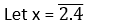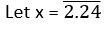# RS Aggarwal Solutions Chapter 1 Real Numbers Exercise - 1C Class 10 Maths

 Chapter Name RS Aggarwal Chapter 1 Real Numbers Solutions Book Name RS Aggarwal Mathematics for Class 10 Other Exercises Exercise 1AExercise 1BExercise 1DExercise 1E Related Study NCERT Solutions for Class 10 Maths

### Exercise 1C Solutions

1. Without actual division, show that each of the following rational numbers is a terminating decimal. Express each in decimal form.

(i) 23/(23 × 52)

(ii) 24/125

(iii) 171/800

(iv) 15/1600

(v) 17/320

(vi) 19/3125

Solution

(i) 23/(23 × 52)

= (23 × 5)/(23 × 53)

= 115/1000

= 0.115

We know either 2 or 5 is not a factor of 23, so it is in its simplest form.

Moreover, it is in the form of (2× 5n).

Hence, the given rational is terminating.

(ii) 24/125

= 24/53

= (24 × 23)/(53 × 23)

= 192/1000

= 0.192

We know 5 is not a factor of 23, so it is in its simplest form. Moreover, it is in the form of (2× 5n).

Hence, the given rational is terminating.

(iii) 171/800

= 171/(25 × 52)

= (171 × 53)/(25 × 55)

= 21375/100000

= 0.21375

We know either 2 or 5 is not a factor of 171, so it is in its simplest form.

Moreover, it is in the form of (2m × 5n).

Hence, the given rational is terminating.

(iv) 15/1600

= 15/(26 × 52)

= (15 × 54)/(26 × 56)

= 9375/1000000

= 0.009375

We know either 2 or 5 is not a factor of 15, so it is in its simplest form.

Moreover, it is in the form of (2m × 5n).

Hence, the given rational is terminating.

(v) 17/320

= 17/(26 × 5)

= (17 × 55)/(26 × 56

= 53125/1000000

= 0.053125

We know either 2 or 5 is not a factor of 17, so it is in its simplest form.

Moreover, it is in the form of (2m × 5n).

Hence, the given rational is terminating.

(vi) 19/3125

= 19/(55)

= (19 × 25)/(25 × 55)

= 608/100000

= 0.00608

We know either 2 or 5 is not a factor of 19, so it is in its simplest form. Moreover, it is in the form of (2m × 5n).

Hence, the given rational is terminating.

2. Without actual division show that each of the following rational numbers is a non terminating repeating decimal.

(i) 11/(23 × 3)

(ii) 73/(23 × 33 × 5)

(iii) 129/(22 × 57 × 75)

(iv) 9/35

(v) 7/210

(vi) 32/147

(vii) 29/343

(viii) 64/455

Solution

(i) 11/(23 × 3)

We know either 2 or 3 is not a factor of 11, so it is in its simplest form.

Moreover, (23 × 3) ≠ (2m × 5n

Hence, the given rational is non–terminating repeating decimal.

(ii) 73/(23 × 33 × 5)

We know 2, 3 or 5 is not a factor of 73, so it is in its simplest form.

Moreover, (22× 33 × 5) ≠ (2m × 5n

Hence, the given rational is non-terminating repeating decimal.

(iii) 129/(22× 57 × 75)

We know 2, 5 or 7 is not a factor of 129, so it is in its simplest form.

Moreover, (22× 57× 75) ≠ (2m × 5n

Hence, the given rational is non-terminating repeating decimal.

(iv) 9/35 = 9/(5 × 7)

We know either 5 or 7 is not a factor of 9, so it is in its simplest form.

Moreover, (5 × 7) ≠ (2m × 5n

Hence, the given rational is non-terminating repeating decimal.

(v) 77/210

= (77 + 7)/(210 + 7)

= 11/30

= 11/(2 × 3 × 5)

We know 2, 3 or 5 is not a factor of 11, so 11/30 is in its simplest form.

Moreover, (2 × 3 × 7) ≠ (2m × 5n

Hence, the given rational is non-terminating repeating decimal.

(vi) 32/147 = 32/(3 × 72)

We know either 3 or 7 is not a factor of 32, so it is in its simplest form.

Moreover, (3 × 72) ≠ (2m × 5n

Hence, the given rational is non-terminating repeating decimal.

(vii) 29/343 = 29/73

We know 7 is not a factor of 29, so it is in its simplest form.

Moreover, 73 ≠ (2m × 5n

Hence, the given rational is non-terminating repeating decimal.

(viii) 64/455 = 64/(5 × 7 × 13)

We know 5, 7 or 13 is not a factor of 64, so it is in its simplest form.

Moreover, (5 × 7 × 13) ≠ (2m × 5n

Hence, the given rational is non-terminating repeating decimal.

3. Express each of the following as a rational number in its simplest form:

(i) 0.8

(ii) 2.4
(iii) 0.24
(iv) 0.12
(v) 2.24
(vi)0.365
Solution
∴ x = 0.888 …(1)

⇒ 10x = 8.888 …(2)

On subtracting equation (1) from (2), we get

9x = 8

⇒ x = 8/9

(ii)∴ x = 2.444 …(1)

10x = 24.444 …(2)

On subtracting equation (1) from (2), we get

9x = 22

⇒ x = 22/9

∴ x = 0.2424 …(1)

100x = 24.2424 …(2)

On subtracting equation (1) from (2), we get

99 x = 24

⇒ x = 8/33

∴ x = 0.1212 …(1)

⇒ 100x = 12.1212 …(2)

On subtracting equation (1) from (2), we get

99x = 12

⇒ x = 4/33

(v)∴ x = 2.2444 …(1)

⇒ 10x = 22.444 …(2)

⇒ 100x = 224.444 …(3)

On subtracting equation (2) from (3), we get

90x = 202

⇒ 202/90 = 101/45

∴ x = 0.3656565 …(1)

⇒ 10x = 3.656565 …(2)

⇒ 1000x = 365.656565 …(3)

On subtracting equation (2) from (3), we get

990x = 362

⇒ x = 362/990 = 181/495